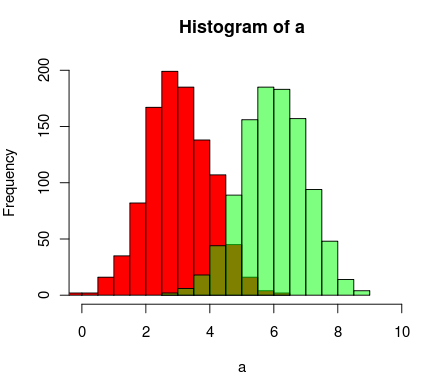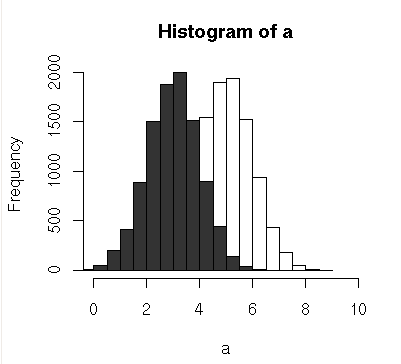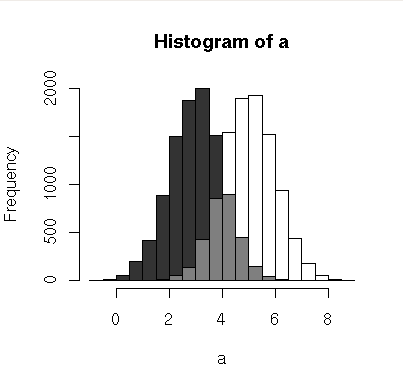## Transparent histograms redux

The other day, I posted an R function that simulates transparency where histograms overlap. In the comments, Alice left this tip:

You could also use transparent colors, which could mean less programming effort:
e.g. col=rgb(0, 1, 0,0.5)) ..the 4th argument is the degree of transparency alpha

*smacks forehead* That’s a lot simpler than my solution. I didn’t know R supported transparency like that. So instead of using the function I wrote, I could have just done something like this:

```a=rnorm(1000, 3, 1) b=rnorm(1000, 6, 1) hist(a, xlim=c(0,10), col="red") hist(b, add=T, col=rgb(0, 1, 0, 0.5)```

And gotten the following output:Much simpler.

## Transparent overlapping histograms in R

Today I wanted to compare the histograms from two data sets, but it’s hard to see the differences when the plots overlap. Here’s an example of the problem:
[sourcecode]
a = rnorm(10000,5)
b = rnorm(10000,3)
hist(a,xlim=c(0,10))
[/sourcecode]
Output:I want to know what’s going on behind all that solid grey!

So I coded up a little function to add pseudo-transparency to pairs of histograms:
[sourcecode]
plotOverlappingHist <- function(a, b, colors=c("white","gray20","gray50"),
breaks=NULL, xlim=NULL, ylim=NULL){

ahist=NULL
bhist=NULL

if(!(is.null(breaks))){
ahist=hist(a,breaks=breaks,plot=F)
bhist=hist(b,breaks=breaks,plot=F)
} else {
ahist=hist(a,plot=F)
bhist=hist(b,plot=F)

dist = ahist\$breaks-ahist\$breaks
breaks = seq(min(ahist\$breaks,bhist\$breaks),max(ahist\$breaks,bhist\$breaks),dist)

ahist=hist(a,breaks=breaks,plot=F)
bhist=hist(b,breaks=breaks,plot=F)
}

if(is.null(xlim)){
xlim = c(min(ahist\$breaks,bhist\$breaks),max(ahist\$breaks,bhist\$breaks))
}

if(is.null(ylim)){
ylim = c(0,max(ahist\$counts,bhist\$counts))
}

overlap = ahist
for(i in 1:length(overlap\$counts)){
if(ahist\$counts[i] > 0 & bhist\$counts[i] > 0){
overlap\$counts[i] = min(ahist\$counts[i],bhist\$counts[i])
} else {
overlap\$counts[i] = 0
}
}

plot(ahist, xlim=xlim, ylim=ylim, col=colors)
}
[/sourcecode]

The results are much easier to interpret:
[sourcecode]
a = rnorm(10000,5)
b = rnorm(10000,3)
plotOverlappingHist(a,b)
[/sourcecode]I’m sure this could be improved upon and generalized to multiple histograms, but this solves my problem, so I’m calling it quits for now. Let me know if you make improvements or find it useful.

|